# Introduction

A description of microbial growth is essential for shelf-life estimation and microbial risk assessment of food products. The biogrowth package for R includes several functions for modelling microbial growth according to the methods developed within the field of predictive microbiology. This includes:

• Model fitting of primary models to data gathered under static conditions.
• Model fitting of secondary (cardinal) models to growth rates gathered under static conditions.
• One-step model fitting of growth models to data gathered under dynamic conditions.
• Deterministic predictions under static or dynamic conditions.
• Stochastic predictions under static or dynamic conditions.

The calculations are done using mathematical models commonly used in predictive microbiology (Perez-Rodriguez and Valero, 2012). Primary modelling is done using the Baranyi, modified Gompertz and trilinear models, whereas secondary modelling is done using the gamma concept.

Once installed, biogrowth can be loaded with

library(biogrowth)

This loads all the relevant functions in the package. Moreover, this also loads the example datasets that are included in the package.

In this document, we will present the functions included in biogrowth. In order to ease the manipulations, we will be using the tidyverse package.

library(tidyverse)

Several functions in the package make use of stochastic algorithms. For reproducibility, we will set the seed of R’s internal random number generator (PRNG) to some arbitrary value

set.seed(1241)

According to the conventions in predictive microbiology, in this document we will use $$log$$ to refer to the decimal logarithm and $$ln$$ for the natural logarithm.

# Mathematical background

## Microbial growth according to predictive microbiology

In predictive microbiology mathematical models are usually divided in primary and secondary models (Perez-Rodriguez and Valero, 2012). Primary models describe the variation of the variable(s) of interest (usually the microbial count) against time. Due to the strong empirical component of these models, primary models have model parameters that are dependent on the environmental conditions. A typical example is the relationship between the specific growth rate and the storage temperature. In contrast, secondary models describe the relationship between the parameters of the primary model and the environmental conditions.

## Primary growth models included in biogrowth

### Modified Gompertz model under static conditions

Zwietering et al. (1990) proposed a reparameterization of the Gompertz model with parameters that have a biological interpretation for microbial growth. This model predicts the microbial count $$N(t)$$ for storage time $$t$$ as a sigmoid using the following algebraic equation

$\log N(t) = \log N_0 + C \left( \exp \left( -\exp \left( 2.71 \frac{\mu}{C}(\lambda-t)+1 \right) \right) \right)$

where $$N_0$$ is the initial microbial count, $$\mu$$ is the maximum specific growth rate, $$\lambda$$ is the duration of the lag phase and $$C$$ is the difference between the initial microbial count and the maximum microbial count.

### Baranyi model under dynamic conditions

Baranyi and Roberts (1994) proposed a system of two differential equations to describe microbial growth:

$\frac{dN}{dt} = \frac{Q}{1+Q}\mu\left(1 - \frac{N}{N_{max}} \right)N$

$\frac{dQ}{dt}=\mu \space Q$ In this model, the deviations with respect to exponential growth are justified based on two hypotheses. It introduces the variable $$Q(t)$$, which represents a theoretical substance that must be produced before the microorganism can enter the exponential growth phase. Hence, its initial value ($$Q_0$$) defines the lag phase duration (under static conditions) as $$\lambda = \frac{\ln (1+1/Q_{0})}{\mu}$$. On the other hand, the stationary growth phase is defined by the logistic term $$(1-N/N_{max})$$, which reduces the growth rate as the microorganisms reach the maximum count.

Note that the original paper by Baranyi and Roberts included an exponent, $$m$$, in the term defining the stationary growth phase. However, that term is usually set to 1 in predictive microbiology and, consequently, has been omitted from biogrowth. Also, in its original paper, the specific growth rate of $$Q(t)$$ was given by a different growth rate ($$\nu$$). However, because this variable does not correspond to any known substance, this growth rate lacks a biological meaning and has been set to $$\mu$$.

### Baranyi model under static conditions

For static conditions, the differential equation proposed by Baranyi and Roberts (1994) has as particular solution the sigmoid given by

$\log N(t) = \log N_0 + \mu \cdot A(t) - \frac{\log(1 + (\exp(\mu \cdot A(t)) - 1)}{ \exp(\log N_{max} - \log N_0))}$ where $$A$$ is an adjustment function:

$A(t) = t + \frac{1}{\mu} \log(\exp(-\mu * t) + \exp(-h_0) - \exp(-\mu \cdot t - h_0))$

In these equations, where $$N_0$$ is the initial microbial count, $$\mu$$ is the maximum specific growth rate and $$N_{max}$$ is the maximum growth rate. The parameter $$h_0$$ is usually called the work to be done and is defined as $$h_{0} = \mu\cdot\lambda$$, where $$\lambda$$ is the lag phase. For compatibility with the other models, the functions in biogrowth take as input $$\lambda$$ instead of $$h_0$$.

### Trilinear model under static conditions

Buchanan et al. (1997) proposed a trilinear model as a more simple approach to describe microbial growth. This model is defined by the piece-wise equation.

The lag phase is defined considering that, as long as $$t < \lambda$$, there is no growth (i.e. $$N = N_0$$).

$\log N(t) = \log N_0; t \leq \lambda$ The exponential phase is described considering that during this phase, the specific growth rate is constant, with slop $$\mu_{max}$$.

$\log N(t) = \log N_0 + \mu(t-\lambda); t\in(\lambda,t_{max})$

Finally, the stationary phase is modelled considering that once $$N$$ reaches $$N_{max}$$, it remains constant.

$\log N(t) = \log N_{max}; t \geq t_{max}$

where $$t_{max}$$, defined is the time required for the microbial count to reach $$N_{max}$$.

### Gathering meta data about primary models directly from biogrowth

The biogrowth package includes the primary_model_data() function, which provides information about the primary models included in the package. It takes just one argument (model_name). By default, this argument is NULL, and the function returns the identifiers of the available models.

primary_model_data()
#>  "modGompertz" "Baranyi"     "Trilinear"

If, instead, one passess to this function any valid model identifier, the function returns the meta-information of that model.

meta_info <- primary_model_data("Trilinear")

That includes the full reference

meta_info$ref #>  "Buchanan, R. L., Whiting, R. C., and Damert, W. C. (1997). When is simple good enough: A comparison of the Gompertz, Baranyi, and three-phase linear models for fitting bacterial growth curves. Food Microbiology, 14(4), 313-326. https://doi.org/10.1006/fmic.1997.0125" or the name of the parameters of this model meta_info$pars
#>  "logN0"   "logNmax" "mu"      "lambda"

## Secondary growth models included in biogrowth

Although other approaches for secondary modeling exist in the literature, the biogrowth package uses the gamma approach proposed by Zwietering et al. (1992). This approach assumes that the effect of each environmental factor on the growth rate is multiplicative and independent. Hence, the specific growth rate ($$\mu$$) observed under a combination of environmental conditions ($$X_1$$, $$X_2$$,…,$$X_n$$) is the result of multiplying the value of $$\mu$$ observed under optimal conditions for growth ($$\mu_{opt}$$) times a gamma factor corresponding to each environmental factor ($$\gamma(X_i)$$). Because gamma factors represent growth rates under sub-optimal conditions, they are correction factors taking values between 0 and 1.

Note that this approach does not take into consideration interaction between the different terms. The reason for this is that, in the opinion of the authors, their implementation is still a research topic, without any model being broadly accepted. Therefore, the inclusion of interactions between terms is not in line with the philosophy of biogrowth, which aims at easing the application of commonly accepted modelling approaches.

$\mu = \mu_{opt} \cdot \gamma(X_1) \cdot \gamma(X_2) \cdot ... \cdot \gamma(X_n)$

### Gamma factors by Zwietering

Zwietering et al. (1992) defined several functions for different environmental factors (temperature, pH and water activity). These functions can be generalized as

$\gamma(X) = \left( \frac{X-X_{min}}{X_{opt}-X_{min}} \right)^n$

where $$X_min$$ is the minimum value of $$X$$ enabling growth, $$X_{opt}$$ is the optimum value of $$X$$ for growth and $$n$$ is the order of the model. The latter value usually takes the value 1 or 2, depending on the environmental factor.

### Cardinal Parameter Model (CPM)

Rosso et al. (1995) defined a piecewise equation for the gamma factors

$\gamma(X)=0; X<X_{min}$

$\gamma(X)=0; X>X_{max}$

$\gamma(X) = \frac{(X-X_{max})(X-X_{min})^n} {(X_{opt}-X_{min})^{n-1}( (X_{opt}-X_{min})(X-X_{opt}) - (X_{opt}-X_{max}) \cdot ((n-1)X_{opt} + X_{min}-n \cdot X) )}; otherwise$ In this equation, $$X_{min}$$, $$X_{opt}$$ and $$X_{max}$$ are the minimum, maximum and optimum values of $$X$$ for microbial growth (usually called cardinal parameters). The coefficient $$n$$ is the shape parameter of the cardinal model.

The full Ratkowsky model (Ratkowsky et al., 1983) is an extension of the square-root model by Ratkowsky (Ratkowsky et al., 1982) that accounts for the decline of the growth rate for temperatures higher than the optimal one. It is described by the following equation

$\sqrt{\mu} = b \left( X-X_{min} \right) \left(1-e^{c(X-X_{max})} \right)$ The application of the gamma concept requires that every gamma function takes values between 0 and 1, whereas the full Ratkowsky model takes values between 0 and $$\sqrt{\mu_{opt}}$$. This can be adapted by defining a gamma function such as

$\gamma_{Ratkowsky}= \left( \frac{\sqrt{\mu}}{ \sqrt{\mu_{opt}} } \right)^2$

For that, we need to know the value of $$\sqrt{\mu_{opt}}$$, which is not included as a parameter in the full Ratkowsky model. By setting the first derivative with respect to $$X$$ to 0, we get to

$X_{opt} = \frac{ W_n \left( e^{-cX_{min} +cX_{max} + 1} \right) + cX_{min} - 1 } {c}$

where $$W_n$$ is the Lambert-W function. Then, the maximum growth rate under optimal conditions is given by

$\sqrt{\mu_{opt}} = b \left( X_{opt}-X_{min} \right) \left(1-e^{c(X_{opt}-X_{max})} \right)$

and the gamma function for the Ratkowsky model can be calculated as

$\gamma_{Ratkowsky}= \left( \frac{\sqrt{\mu}}{ \sqrt{\mu_{opt}} } \right)^2 = \left( \frac{ b \left( X-X_{min} \right) \left(1-e^{c(X-X_{max})} \right)} { b \left( X_{opt}-X_{min} \right) \left(1-e^{c(X_{opt}-X_{max})} \right)} \right)^2 = \left( \frac{ \left( X-X_{min} \right) \left(1-e^{c(X-X_{max})} \right)} {\left( X_{opt}-X_{min} \right) \left(1-e^{c(X_{opt}-X_{max})} \right)} \right)^2$

Note that parameter $$b$$ banishes after doing the division, so this model has 3 model parameters: $$c$$, $$X_{min}$$ and $$X_{max}$$.

### Gathering meta data about secondary models directly from biogrowth

The biogrowth package includes the secondary_model_data() function, which provides information about the primary models included in the package. It takes just one argument (model_name). By default, this argument is NULL, and the function returns the identifiers of the available models.

secondary_model_data()
#>  "CPM"           "Zwietering"    "fullRatkowsky"

If, instead, one passess any valid model identifier, the function returns its meta-information.

meta_info <- secondary_model_data("CPM")

This includes the full reference of the model

meta_info$ref #>  "Rosso, L., Lobry, J. R., Bajard, S., and Flandrois, J. P. (1995). Convenient Model To Describe the Combined Effects of Temperature and pH on Microbial Growth. Applied and Environmental Microbiology, 61(2), 610-616." or the names of its model parameters meta_info$pars
#>  "xmin" "xopt" "xmax" "n"

## Numerical methods

The numerical calculations are largely based on the FME and deSolve packages. The differential equations required for dynamic calculations are calculated using the ode() function from deSolve, which implements the LSODA numerical method. Model fitting is done using the modFit() function from FME for linear regression and the modMCMC function from the same package for MCMC fitting.

# Datasets included in the package

The biogrowth package includes three datasets to aid in the understanding of its functions: * example_cardinal: an example of the results of a set of growth experiments to estimate cardinal parameters. * example_dynamic_growth and example_env_conditions: an example of the results of a dynamic growth experiment.

All of them are automatically saved when the package is installed. Each one of them can be loaded with a call to the function data() passing the name of the dataset as an argument.

The dataset example_cardinal includes an example of the type of data used for estimating cardinal model parameters. It has three columns: temperature, pH and mu. The two first represent the storage conditions during several static growth experiments, whereas the latter is the specific growth rate estimated in those experiments. This dataset is intended to be used for fit_secondary_growth().

data("example_cardinal")
#>   temperature pH           mu
#> 1    0.000000  5 9.768505e-04
#> 2    5.714286  5 2.624919e-03
#> 3   11.428571  5 0.000000e+00
#> 4   17.142857  5 1.530706e-04
#> 5   22.857143  5 2.301817e-05
#> 6   28.571429  5 3.895598e-04

The datasets example_dynamic_growth and example_env_conditions describe a dynamic growth experiment, which can be used for the fit_dynamic_growth() function. The dataset example_env_conditions describes the experimental design; i.e. how the environmental factors vary during the experiment. It has three columns: time (the elapsed time), temperature (the storage temperature) and aw (the water activity).

data("example_env_conditions")
#> # A tibble: 3 x 3
#>    time temperature    aw
#>   <dbl>       <dbl> <dbl>
#> 1     0          20  0.99
#> 2     5          30  0.95
#> 3    15          35  0.9

The dataset example_dynamic_growth illustrates the microbial counts observed during the experiment described by example_env_conditions. It has two columns: time (the elapsed time) and logN (the observed microbial count).

data("example_dynamic_growth")
#> # A tibble: 6 x 2
#>    time     logN
#>   <dbl>    <dbl>
#> 1 0      0.0670
#> 2 0.517 -0.00463
#> 3 1.03  -0.0980
#> 4 1.55  -0.0986
#> 5 2.07   0.111
#> 6 2.59  -0.0465

The dataset multiple_experiments simulates several growth experiments performed for the same microorganism under dynamic conditions that vary between experiments. It is a nested list with two elements, each describing a single experiment.

data("multiple_experiments")
length(multiple_experiments)
#>  2

Each experiment is described using a list with two elements: data and conditions. The former is a tibble with two columns: time (the elapsed time) and logN the observed microbial count.

head(multiple_experiments[]$data) #> # A tibble: 6 x 2 #> time logN #> <dbl> <dbl> #> 1 0 -0.208 #> 2 1.67 -0.0363 #> 3 3.33 -0.298 #> 4 5 0.350 #> 5 6.67 0.143 #> 6 8.33 -0.404 Then, conditions is also a tibble with one column named time (elapsed time), one called temperature (the storage temperature) and another one called pH (the pH of the media). print(multiple_experiments[]$conditions)
#> # A tibble: 3 x 3
#>    time temperature    pH
#>   <dbl>       <dbl> <dbl>
#> 1     0          20   6.5
#> 2    15          30   7
#> 3    40          40   6.5

The second experiment is described using the same structure.

head(multiple_experiments[]$data) #> # A tibble: 6 x 2 #> time logN #> <dbl> <dbl> #> 1 0 0.533 #> 2 1.67 -0.336 #> 3 3.33 0.0650 #> 4 5 -0.121 #> 5 6.67 0.165 #> 6 8.33 -0.0198 print(multiple_experiments[]$conditions)
#> # A tibble: 3 x 3
#>    time temperature    pH
#>   <dbl>       <dbl> <dbl>
#> 1     0          25     7
#> 2    15          30     6
#> 3    40          25     5

# Deterministic modelling

## Growth prediction under static conditions

The biogrowth package includes the function predict_isothermal_growth to make predictions under static conditions. The calculations are based on the primary model, without the need of defining a secondary model. This function has 3 arguments:

• model_name: a character vector indicating the primary model. Admisible values are those returned by primary_model_data.
• times: a numeric vector of storage times to make the predictions.
• model_pars: a named list defining the values of the model parameters.

For instance, to make predictions using the modified Gompertz model we would define

my_model <- "modGompertz"

This model has 3 model parameters: mu, lambda and C (retrieved using primary_model_data). Additional, for making the calculations, we need to define an initial microbial count (logN0). All this information must be defined in a single list:

my_pars <- list(logN0 = 2, C = 6, mu = .2, lambda = 25)

Finally, we have to define the storage times for which the prediction is calculated. For instance, we can define

my_time <- seq(0, 100, length = 1000)

Once we have defined the arguments, we can call the function predict_isothermal_growth to get the model predictions. This function automatically checks that the number of model parameters and their names and raises a warning or error if there are mismatches between the arguments and the model definition.

static_prediction <- predict_isothermal_growth(my_model, my_time, my_pars)

This function returns a list of class IsothermalGrowth with the results of the simulation. It has three items:

• simulation: A tibble with the results of the simulation.
• model: The name of the model used for making the calculations.
• pars: Vector of model parameters used for the calculations.

Hence, we can retrieve the results of the simulation by accessing the simulation item

static_prediction$simulation #> # A tibble: 1,000 x 2 #> time logN #> <dbl> <dbl> #> 1 0 2. #> 2 0.100 2. #> 3 0.200 2. #> 4 0.300 2. #> 5 0.400 2. #> 6 0.501 2. #> 7 0.601 2. #> 8 0.701 2. #> 9 0.801 2. #> 10 0.901 2. #> # … with 990 more rows Alternatively, this class implements an S3 method for plot that illustrates the survivor curve: plot(static_prediction)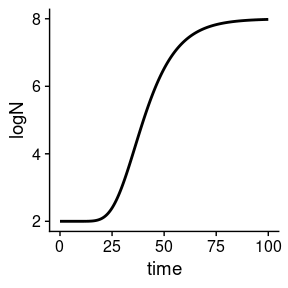The plot is generated as a gpplot object, with theme_cowplot() as default. Therefore, it can be edited using layers as usual in the ggplot2 package. For instance, plot(static_prediction) + xlab("Storage time (h)") + ylab("Microbial count (log CFU/ml)") + theme_gray()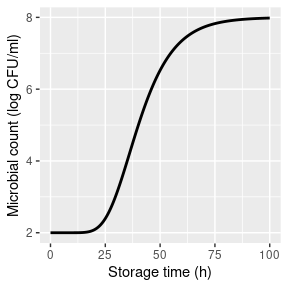See the vignette #ADD LINK HERE for pretty graphics ## Growth prediction under dynamic conditions The biogrowth package can also be used for simulating growth under dynamic conditions using the predict_dynamic_growth() function. This function has 5 arguments: • times: Numeric vector of storage times to make the calculations. • env_conditions: A tibble describing the variation of the environmental conditions through storage. • primary_pars: A list describing the model parameters of the primary model. • secondary_models: A nested list defining the secondary model(s). • ...: Additional arguments passed to the numeric solver of the differential equation. The varying environmental conditions are defined using a tibble. This object must have a column named time and as many additional columns as needed for each environmental factor. For the simulations, the value of the environmental conditions at time points not included in env_conditions is calculated by linear interpolation. For instance, we can define the temperature and pH variation through storage using the following tibble: my_conditions <- tibble(time = c(0, 5, 40), temperature = c(20, 30, 35), pH = c(7, 6.5, 5) ) Then, the simulations would consider this temperature profile ggplot(my_conditions) + geom_line(aes(x = time, y = temperature))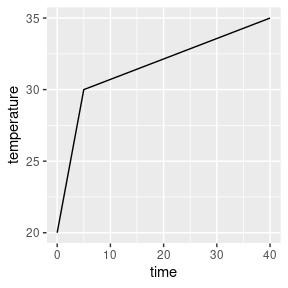And this pH profile ggplot(my_conditions) + geom_line(aes(x = time, y = pH))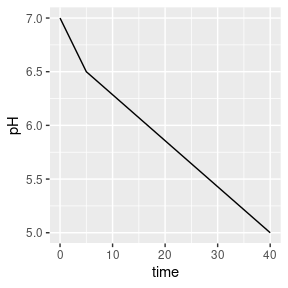Therefore, in order to get smoother profiles, one has to define additional rows in the tibble. For dynamic conditions, biogrowth can only use the Baranyi growth model as primary model. This model requires the definition of two model parameters: the specific growth rate at optimum conditions (mu_opt) and the maximum microbial count (Nmax). Moreover, the initial values of the microbial count (N0) and the theoretical substance $$Q$$ (Q0) must be defined. Note that $$Q_0$$ is related to the duration of the lag phase under isothermal conditions by the identity $$\lambda = \ln \left( 1 + 1/q_0 \right)/\mu_{max}$$. For the predict_dynamic_growth()function, they all must be defined in a single list: my_primary <- list(mu_opt = 2, Nmax = 1e8, N0 = 1e0, Q0 = 1e-3) The next step is the definition of the secondary models. As already described above, biogrowth describes the variation of $$\mu$$ with temperature based on the gamma concept. Therefore, we need to define one secondary model per environmental condition. This must be done using a list. We define a list per environmental condition and this list defines the type of gamma model as well as the model parameters. The function secondary_model_data() can aid in the definition of the secondary models. For instance, we will define a gamma-type model for temperature as defined by Zwietering et al. This is done by including an item called model in the list and assigning it the value "Zwietering". Then, we define the values of the model parameters. In this case, we need the minimum (xmin) and optimum (xopt) cardinal values, as well as the order of the model (n) (this information can be retrieved using secondary_model_data). We define them using individual entries in the list: sec_temperature <- list(model = "Zwietering", xmin = 25, xopt = 35, n = 1) Next, we will define a CPM model for the effect of pH. Note that the model selection is for illustration purposes, not based on predictive power. The definition is very similar as for the Zwietering-type model. First of all, we need to set the item model to "CPM" instead of "Zwietering". Then, we need to give one additional parameter value (xmax): sec_pH = list(model = "CPM", xmin = 5.5, xopt = 6.5, xmax = 7.5, n = 2) The final step for the definition of the gamma-type secondary model is putting all the individual models together in a single list. Be mindful, though, that every element on the list must be named using the same column names as in env_conditions. Before, we had used the column names temperature and pH. Therefore, we must repeat the exact same names in the definition of the secondary model. my_secondary <- list( temperature = sec_temperature, pH = sec_pH ) The final argument is the time points where to make the calculations. We can use a numeric vector between 0 and 50 for this: my_times <- seq(0, 50, length = 1000) Once we have defined every argument, we can call the predict_dynamic_growth() function: dynamic_prediction <- predict_dynamic_growth(my_times, my_conditions, my_primary, my_secondary) This function returns a list of class DynamicGrowth with several items: • simulation: A tibble with the results of the simulation. • gammas: A tibble describing the variation of each gamma factor through the simulation. • env_conditions: Environmental conditions used for the simulations. • primary_pars: Primary model parameters used for the simulations. • sec_models: Secondary model parameters used for the simulations. Therefore, the results of the simulation can be retrieved from the simulation item: dynamic_prediction$simulation
#> # A tibble: 1,000 x 4
#>      time     Q     N  logN
#>     <dbl> <dbl> <dbl> <dbl>
#>  1 0      0.001     1     0
#>  2 0.0501 0.001     1     0
#>  3 0.100  0.001     1     0
#>  4 0.150  0.001     1     0
#>  5 0.200  0.001     1     0
#>  6 0.250  0.001     1     0
#>  7 0.300  0.001     1     0
#>  8 0.350  0.001     1     0
#>  9 0.400  0.001     1     0
#> 10 0.450  0.001     1     0
#> # … with 990 more rows

Alternatively, this class implements an S3 method for plot that can be used to visualize the growth curve:

plot(dynamic_prediction)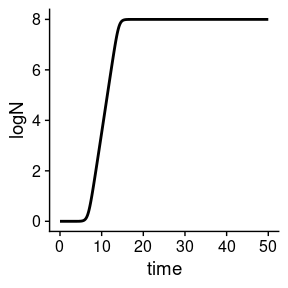The argument add_factor of the plot method can be used to plot the variation of a single environmental factor through storage. For that, one has to pass the name of the desired factor to the function. Note that this name must be identical to the one used for the columns in env_conditions. For instance, we can add the plot of temperature

plot(dynamic_prediction, add_factor = "temperature")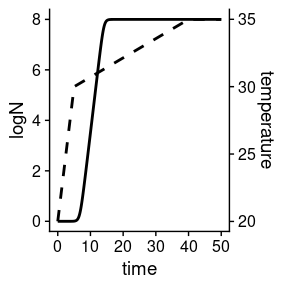The arguments ylims, label_y1 and label_y2 enable to further edit the plot by changing the limits of the y-axis and the names of the primary and secondary axes:

plot(dynamic_prediction, add_factor = "temperature", ylims= c(0, 8),
label_y1 = "Microbial count (log CFU/ml)", label_y2 = "Storage temperature (ºC)")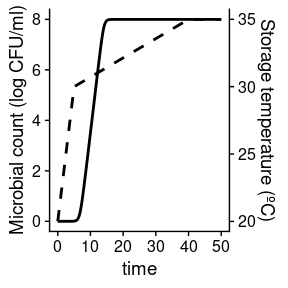## Time to reach a given microbial count

For shelf-life estimation and food safety, it is usually of interest to calculate the storage time required to reach a given microbial count. The biogrowth package includes the function time_to_logcount() can be used for this purpose. This function has 2 arguments:

• model: A model returned by predict_dynamic_growth() or predict_isothermal_growth().
• log_count: target microbial count.

For instance, we can use this function to estimate the time required to reach a microbial count of 2.5 log CFU/ml for the static prediction we did earlier:

time_to_logcount(static_prediction, 2.5)
#>  25.99053

Or the time required to reach 5 log CFU/ml in the dynamic prediction:

time_to_logcount(dynamic_prediction, 5)
#> Warning in regularize.values(x, y, ties, missing(ties)): collapsing to unique
#> 'x' values
#>  11.36447

If the value of log_count was outside the range of the simulations, the function returns an NA:

time_to_logcount(dynamic_prediction, 10)
#> Warning in regularize.values(x, y, ties, missing(ties)): collapsing to unique
#> 'x' values
#>  NA

Note that the calculations are based on linear interpolation of the simulated growth curve. Therefore, its accuracy is strongly dependent on the number of time points used for the simulation.

## Fitting of primary models

The function fit_isothermal_growth() can be used to estimate the values of primary growth models from data obtained under static conditions. This function has 4 arguments:

• fit_data defines the data used for model fitting.
• model_name defines the primary model to use (according to primary_model_data()).
• starting_point defines the initial values of the model parameters (according to primary_model_data()).
• known_pars defines parameters that are considered known and are not fitted to the data.
• ... can be used to pass additional arguments to the modFit() function from the FME package (e.g. lower and upper bounds for the model parameters).

The data used for model fitting is defined through the fit_data argument. It must be a tibble with two columns named time (the elapsed time) and logN (the microbial count).

my_data <- tibble(time = c(0, 25, 50, 75, 100),
logN = c(2, 2.5, 7, 8, 8))

The primary model is defined using model_name. For instance, we can use the Baranyi model in this example:

my_model <- "Baranyi"

This function uses non-linear regression (through the modFit() function of the FME package), so it requires initial values for every model parameter to fit. In the case of the Baranyi model, the model parameters are: mu, lambda and logNmax. Additional, the initial microbial count must be defined. Nonetheless, the fit_isothermal_growth() function enables to fix any model parameter before model fit using the known_pars argument. For instance, we can fix the specific growth rate to 0.2

known <- c(mu = .2)

And fit the remaining model parameters

start = c(logNmax = 8, lambda = 25, logN0 = 2)

Once every model parameter has been defined, we can call the fit_isothermal_growth() function:

static_fit <- fit_isothermal_growth(my_data, my_model,
start, known
)

This function returns a list of class FitIsoGrowth with several items:

• data: data used for model fitting
• model: name of the primary model
• starting_point: starting point used for model fitting
• known: fixed model parameters
• fit: object returned by modFit()
• best_prediction: an instance of IsothermalGrowth corresponding to the fitted model.

The FitIsoGrowth class includes several S3 methods to visualize the results. The statistical information of the fit can be retrieved using summary()

summary(static_fit)
#>
#> Parameters:
#>         Estimate Std. Error t value Pr(>|t|)
#> logNmax  7.99600    0.06980  114.56 7.62e-05 ***
#> lambda  24.64154    0.74220   33.20 0.000906 ***
#> logN0    2.05084    0.09201   22.29 0.002007 **
#> ---
#> Signif. codes:  0 '***' 0.001 '**' 0.01 '*' 0.05 '.' 0.1 ' ' 1
#>
#> Residual standard error: 0.09879 on 2 degrees of freedom
#>
#> Parameter correlation:
#>         logNmax  lambda   logN0
#> logNmax 1.00000 0.05788 0.01589
#> lambda  0.05788 1.00000 0.76437
#> logN0   0.01589 0.76437 1.00000

whereas plot() compares the data and the fitted curve

plot(static_fit)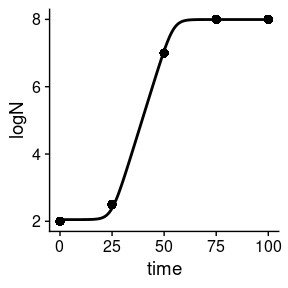## Fitting of secondary (gamma) models

The function fit_secondary_growth() can be used to estimate the values of the cardinal parameters from the specific growth rates observed in a series of static growth experiments. This function has 6 arguments:

• fit_data: data used for the fit.
• starting_point: initial value of the model parameters
• known_pars: model parameters fixed (not fitted to the data).
• sec_model_names: type of secondary model for each environmental factor.
• transformation: transformation of the growth rate for the fit (square root by default).
• ...: additional arguments passed to modFit().

A method to estimate the cardinal parameters is based on realizing various static growth experiments under a variety of experimental conditions. Such dataset is passed using the fit_data argument. The biogrowth package includes an example dataset with the right format.

data("example_cardinal")
#>   temperature pH           mu
#> 1    0.000000  5 9.768505e-04
#> 2    5.714286  5 2.624919e-03
#> 3   11.428571  5 0.000000e+00
#> 4   17.142857  5 1.530706e-04
#> 5   22.857143  5 2.301817e-05
#> 6   28.571429  5 3.895598e-04

This dataset must have one column named mu with the observed growth rate. Then, it must have as many additional columns as environmental factors included in the experiment. In the example dataset, the series of experiments considered two environmental conditions: temperature and pH. Nonetheless, the fit_secondary_growth() function is entirely flexible with respect to the number of factors and their names. The only restriction is that the definition of the columns of the dataset and the secondary models is consistent.

The type of secondary model to use for each environmental factor is defined in the sec_model_names argument. It is a named vector whose names are the environmental factors and whose values define the model to use. The list of available models can be retrieved using secondary_model_data(). For this example, we will use a CPM for the pH and an Zwietering model for temperature (this decision is not based on any scientific argument, just as demonstration of the functions in the package). Note that the names of the vector are identical to the column names of fit_data.

sec_model_names <- c(temperature = "Zwietering",
pH = "CPM")

The fit_secondary_growth() function estimates the values of the cardinal parameters, as well as the specific growth rate under optimal conditions. Also, it enables to fix any model parameter to an arbitrary value before fitting the model. The model parameters to fit are defined using the known_pars argument. The remaining parameters, because of the use of non-linear regression for parameter estimation, require the definition of initial parameter values.

The specific growth rate under optimal conditions is named mu_opt. The remaining cardinal parameters are named according to the convention environ_factor+_+parameter(lower case). For instance, the minimum temperature for growth is temperature_xmin and the order of the CPM for pH is pH_n. Note that the environmental factor must be identical to the one used in sec_model_names.

For this example, we will consider that the maximum specific growth rate is known, as well as most of the the cardinal parameters for pH. For temperature, we will only fix the order of the model.

known_pars <- list(mu_opt = 1.2,
temperature_n = 1,
pH_n = 2, pH_xmax = 6.8, pH_xmin = 5.2
)

The remaining model parameters will be fitted using reasonable initial values.

my_start <- list(temperature_xmin = 5, temperature_xopt = 35,
pH_xopt = 6.5)

Finally, the transformation argument defines the transformation of the growth rate to use for model fitting. By default, the function applies a square root transformation, which has proved to stabilize the variance of microbial growth. Once the arguments have been defined, we can call the fit_secondary_growth() function. Note that, because we are using the default value of transformation, we do not need to define this argument:

fit_cardinal <- fit_secondary_growth(example_cardinal, my_start, known_pars, sec_model_names)

This function returns an instance of FitSecondaryGrowth with 5 items:

• fit_results the object returned by modFit.
• secondary_model parameters of the secondary model.
• mu_opt_fit growth rate under optimal storage conditiosn.
• data data used for the fit.
• transformation tranformation applied to the growth rate before fitting.

This class incorporates several S3 method to ease visualization of results. The function summary() returns the statistical information of the fit.

summary(fit_cardinal)
#>
#> Parameters:
#>                  Estimate Std. Error t value Pr(>|t|)
#> temperature_xmin  5.31768    0.14965   35.53   <2e-16 ***
#> temperature_xopt 34.29640    0.76253   44.98   <2e-16 ***
#> pH_xopt           6.51109    0.01171  555.90   <2e-16 ***
#> ---
#> Signif. codes:  0 '***' 0.001 '**' 0.01 '*' 0.05 '.' 0.1 ' ' 1
#>
#> Residual standard error: 0.04026 on 61 degrees of freedom
#>
#> Parameter correlation:
#>                  temperature_xmin temperature_xopt    pH_xopt
#> temperature_xmin        1.000e+00          -0.1911 -2.376e-09
#> temperature_xopt       -1.911e-01           1.0000 -2.173e-01
#> pH_xopt                -2.376e-09          -0.2173  1.000e+00

The package includes S3 methods to plot the results. By default, a plot comparing observed and predicted values is shown

plot(fit_cardinal)
#> geom_smooth() using formula 'y ~ x'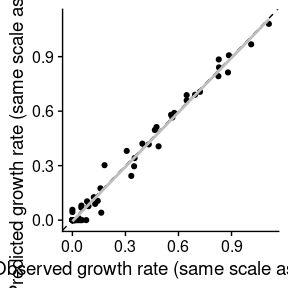In this plot, the dashed line is the line with intercept 0 and slope 1 where every point should fall in case of a perfect fit. The solid grey line is the regression line of predictions vs observations.

Alternativelly, by passing the argument which=2, one can plot the observed and predicted counts as a function of the environmental factors

plot(fit_cardinal, which = 2)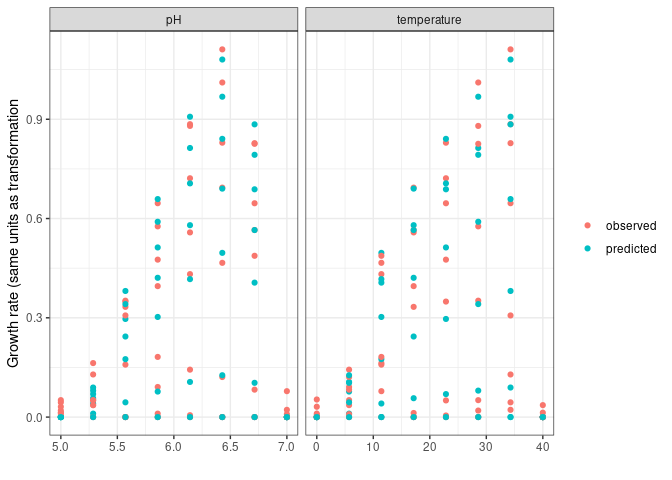A trend line can be added to this plot using the add_trend=TRUE argument:

plot(fit_cardinal, which = 2, add_trend = TRUE)
#> geom_smooth() using method = 'loess' and formula 'y ~ x'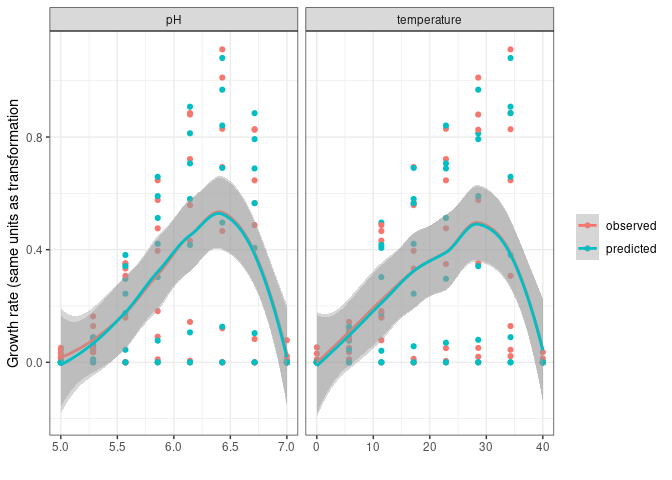Note that this line is not the predictions of the gamma model but a trend line based on the observations. Therefore, it can be used to compare the tendency of the model predictions against the one of the observations, but it should not be extrapolated for predictions.

## One-step fitting under dynamic conditions

The function fit_dynamic_growth() can be used to estimate the parameters of both the primary and the secondary model based on a growth experiment obtained under dynamic conditions. This function has 6 arguments:

• fit_data: data used for model fitting.
• env_conditions: variation of the environmental conditions during the experiment.
• starting_point: initial value of the model parameters.
• known_pars: model parameters known (i.e. not fitted).
• sec_model_names: type of secondary model for each environmental factor.
• ...: additional arguments passed to modFit.

The arguments env_conditions and fit_data are tibbles that describe, respectively, the experimental design and the observations. To help understanding fit_dynamic_growth(), biogrowth includes two example datasets that can be used with this function:

data("example_dynamic_growth")
data("example_env_conditions")

The tibble passed to the argument env_conditions must have a column named time and as many additional columns as environmental factors. The fit_dynamic_growth() function is totally flexible with respect to the number of factors or the way they are named. The only requirement is that the definition of every argument is consistent. In the case of example_env_conditions, this dataset considers two factors: temperature and water activity (aw).

head(example_env_conditions)
#> # A tibble: 3 x 3
#>    time temperature    aw
#>   <dbl>       <dbl> <dbl>
#> 1     0          20  0.99
#> 2     5          30  0.95
#> 3    15          35  0.9

Note that for the calculations this function joins the data points by linear interpolation, as shown in this plot:

ggplot(example_env_conditions, aes(x = time, y = temperature)) +
geom_line() +
geom_point()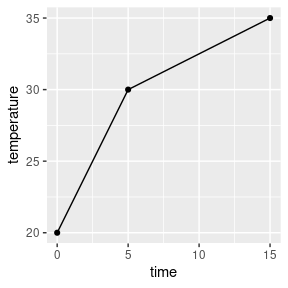In order to have a stepwise profile, one should define additional time points.

The tibble passed to the argument fit_data must have two columns named time (elapsed time) and logN (log microbial count), in the same format as example_dynamic_growth.

head(example_dynamic_growth)
#> # A tibble: 6 x 2
#>    time     logN
#>   <dbl>    <dbl>
#> 1 0      0.0670
#> 2 0.517 -0.00463
#> 3 1.03  -0.0980
#> 4 1.55  -0.0986
#> 5 2.07   0.111
#> 6 2.59  -0.0465

The type of secondary model for each environmental factor is defined by the argument sec_model_names. As in every function in biogrowth, this argument is a named vector where the names refer to the environmental factor and the value the type of model. Supported models can be retrieved using secondary_model_data(). In this example we will use cardinal models for both environmental factors. Note that the names of this vector must be identical to the columns in env_conditions.

sec_model_names <- c(temperature = "CPM",
aw= "CPM")

The fit_dynamic_growth() function enables to fit or fix any model parameter. This distinction is made using the arguments known_pars (fixed parameters) and starting_point (fitted parameters). Note that these arguments must contain every parameter of the primary and secondary models.

Regarding the primary model, this function only enables the use of the Baranyi model. This primary model has two variables that need initial values (N0 and Q0) and one primary model parameter (Nmax). The specific growth rate is described using the gamma concept. This requires the definition of its value under optimal conditions (mu_opt) as well as the cardinal parameters for each environmental factor. As well as for other functions, these must be defined as factor+_+parameter name. For instance, the minimum water activity for growth must be written as aw_min.

In this example we will consider the model parameters of the primary model as known. For the secondary model for water activity, we will only consider the optimum value for growth as unknown. Finally, for the effect of temperature, we will consider the order and xmax are known:

known_pars <- list(Nmax = 1e4,  # Nmax for primary model
N0 = 1e0, Q0 = 1e-3,  # Initial values of the primary model
mu_opt = 4, # mu_opt of the gamma model
temperature_n = 1, temperature_xmax = 40,  # Secondary model for temperature
aw_xmax = 1, aw_xmin = .9, aw_n = 1  # Secondary model for water activity
)

Then, the remaining model parameters must be defined in starting_points. Due to the use of non-linear regression for model fitting, it is required to define initial values for these parameters. They can be defined based on previous esperience or preliminary numerical simulations.

my_start <- list(temperature_xmin = 25, temperature_xopt = 35,
aw_xopt = .95)

Once every model parameter has been defined, we can call the fit_dynamic_growth() function.

my_dyna_fit <- fit_dynamic_growth(example_dynamic_growth, example_env_conditions,
my_start,
known_pars,
sec_model_names)

This function returns an instance of FitDynamicGrowth with 6 items:

• fit_results: object returned by modFit().
• best_prediction: an instance of DynamicGrowth with the prediction corresponding to the fitted model.
• data: data used to fit the model.
• starting: starting values used for parameter estimation.
• known: model parameters considered known.
• sec_models: type of secondary model for each environmental factor.

The FitDynamicGrowth includes several S3 methods that ease the visualization of the results. The summary function returns the statistical information of the fit.

summary(my_dyna_fit)
#>
#> Parameters:
#>                  Estimate Std. Error t value Pr(>|t|)
#> temperature_xmin 26.43224    3.46652   7.625 3.35e-08 ***
#> temperature_xopt 33.85365   10.89999   3.106  0.00443 **
#> aw_xopt           0.99147    0.05574  17.786  < 2e-16 ***
#> ---
#> Signif. codes:  0 '***' 0.001 '**' 0.01 '*' 0.05 '.' 0.1 ' ' 1
#>
#> Residual standard error: 0.1478 on 27 degrees of freedom
#>
#> Parameter correlation:
#>                  temperature_xmin temperature_xopt aw_xopt
#> temperature_xmin           1.0000          -0.9897  0.9762
#> temperature_xopt          -0.9897           1.0000 -0.9971
#> aw_xopt                    0.9762          -0.9971  1.0000

The plot() method compares the model predictions against the data used for the fit:

plot(my_dyna_fit)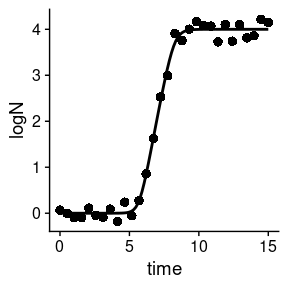The variation of the environmental factor can be plotted alongside the previous plot. For that, the name of the environmental factor must be passed to add_factor. Note that the value passed must be identical to the one defined in the previous arguments. Then, the label of the primary or secondary axes can be changed using label_y1 or label_y2

plot(my_dyna_fit, add_factor = "aw",
label_y1 = "Log count (log CFU/ml)",
label_y2 = "Water activity")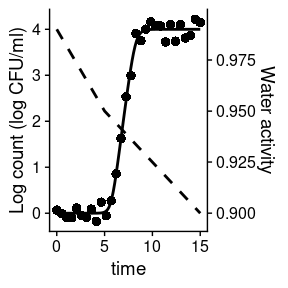## Global fitting of various (dynamic) experiments

The function fit_multiple_growth() can be used to fit one growth model to data gathered in various experiments performed under dynamic conditions. It has several arguments:

• starting_point starting values of the model parameters to fit.
• experiment_data a nested list describing the experimental data and conditions.
• known_pars vector of parameters that are fixed (i.e. not fitted).
• sec_model_names definition of the secondary models for each condition.
• ... additional arguments passed to FME::modFit.

The data to use for the fit is described using the experiment_data argument. It is a nested list with as many elements as experiments. The dataset multiple_experiments serves as an example with the format required by this function.

data("multiple_experiments")

Each experiemnt is described using a list with two elements: data and conditions. The element data is a tibble with two columns, time and logN, that describe the observed log-microbial count.

ggplot(multiple_experiments[]$data) + geom_point(aes(x = time, y = logN))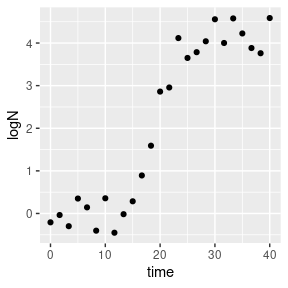The element conditions describes the (dynamic) environmental conditions during storage. It must have one column named time and as many additional columns as environmental conditions considered in the model. Note that, for every simulation, the values of environmental conditions at times not included in the data frame are calculated by linear interpolation.  multiple_experiments[]$conditions %>%
pivot_longer(-time, names_to = "condition", values_to = "value") %>%
ggplot() +
geom_line(aes(x = time, y = value)) +
facet_wrap("condition", scales = "free")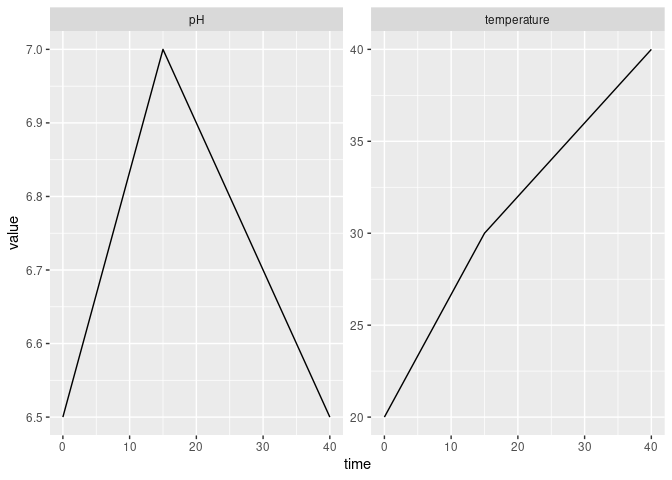The secondary models to use to describe the influence of the environmental conditions on the growth rate is defined using sec_model_names. This argument is a named vector whose names are identical to those in the experimental data and whose values corresponds to valid identifiers according to seccondary_model_data(). For this example, we will use a CPM model for both pH and temperature (for no particular scientific reason).

sec_names <- c(temperature = "CPM", pH = "CPM")

The next step is the definition of what model parameters to estimate from the data and which ones to keep fixed. This is done using the starting_point and known_pars arguments, which are lists. Every parameter of both the primary and secondary models must be included in either of these arguments. The format for parameter definition is identical to the one of fit_dynamic_inactivation.

For this example, we will only fit the maximum specific growth rate and the optimum temperature for growth (for no particular scientific reason).

known <- list(Nmax = 1e8, N0 = 1e0, Q0 = 1e-3,
temperature_n = 2, temperature_xmin = 20, temperature_xmax = 35,
pH_n = 2, pH_xmin = 5.5, pH_xmax = 7.5, pH_xopt = 6.5)

start <- list(mu_opt = .8, temperature_xopt = 30)

Once every argument has been defined, we can call the fit_multiple_growth() function.

global_fit <- fit_multiple_growth(start, multiple_experiments, known, sec_names)

This function returns a list of class FitMultipleDynamicGrowth with several elements. The parameter estimates can be accessed using the S3 method for summary.

summary(global_fit)
#>
#> Parameters:
#>                  Estimate Std. Error t value Pr(>|t|)
#> mu_opt            0.54196    0.01222   44.35   <2e-16 ***
#> temperature_xopt 30.62396    0.18728  163.52   <2e-16 ***
#> ---
#> Signif. codes:  0 '***' 0.001 '**' 0.01 '*' 0.05 '.' 0.1 ' ' 1
#>
#> Residual standard error: 0.4282 on 48 degrees of freedom
#>
#> Parameter correlation:
#>                  mu_opt temperature_xopt
#> mu_opt           1.0000           0.8837
#> temperature_xopt 0.8837           1.0000

Moreover, the predictions of the fitted model can be compared against the data using the S3 method for plot.

plot(global_fit)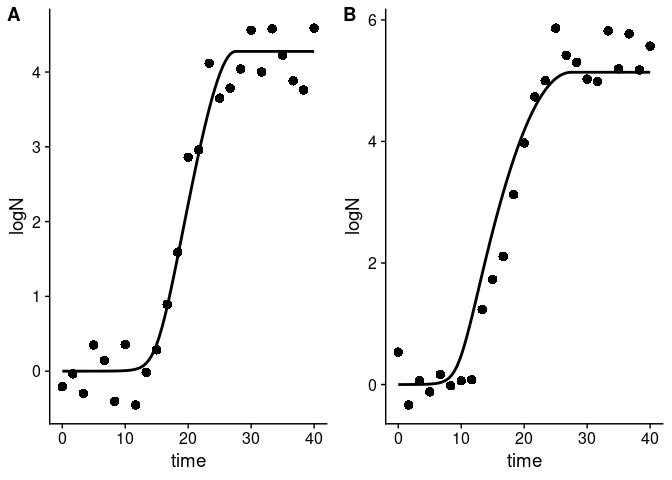This function generates an individual plot for each experiment. Any environmental factor can be included in the plot by passing the name of the factor to the add_factor argument. Note that the name must be identical to the one used for model definition.

plot(global_fit, add_factor = "temperature")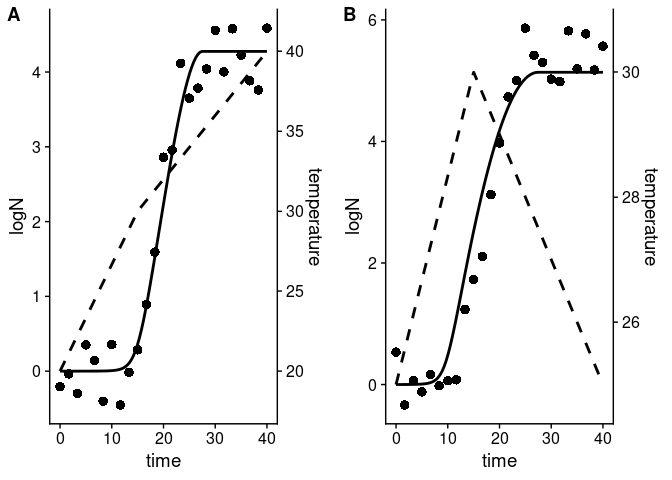# Stochastic modelling

## Stochastic prediction under static conditions

Due to the relevance of uncertainty and variability Quantitative Microbial Risk Assessment usually requires the calculation of stochastic simulations. The function predict_stochastic_growth enables to constract credible intervals of the microbial growth for a normal distribution of: * the square root of $$\mu$$ * the square root of $$\lambda$$ * the logarithm of the initial microbial count * the logarithm of the maximum microbial count

For that, this function includes two arguments per growth parameter (expected value and standard deviation) and 4 additional arguments:

• model_name defines the primary growth model
• times defines the time points where to make the calculations
• n_sims defines the number of Monte Carlo simulations.
• corr_matrix correlation matrix between the model parameters. By default, this argument is set to a diagonal matrix (i.e. no correlation between parameters).

The calculations are done by taking a sample of size n_sims of the model parameters according to a multi-variate normal distribution. For simulation, the microbial growth is predicted and the quantiles of the predicted microbial count is used as an estimate of the credible interval.

For this example, we will use the tri-linear growth model

my_model <- "Trilinear"

For the time points, we will take 100 points uniformly distributed between 0 and 30:

my_times <- seq(0, 30, length = 100)

For the example, we will set the number of simulations to 1000. Nevertheless, it is advisable to repeat the calculations for various number of simulations to ensure that the relevant variables converged.

n_sims <- 1000

Once the arguments have been defined, we can call the predict_stochastic_growth() function.

stoc_growth <- predict_stochastic_growth(my_model, my_times, n_sims,
mean_logN0 = 0, sd_logN0 = .2,
mean_sqmu = 2,sd_sqmu = .3,
mean_sqlambda = 4, sd_sqlambda = .4,
mean_logNmax = 6, sd_logNmax = .5)

This function returns an instance of StochasticGrowth with several items:

• sample: sample of model parameters used for the simulations
• simulations: results of the individual simulations
• quantiles: quantiles of the microbial count predicted in the simulatinos
• model: model used for the simulations
• mus: expected values of the model parameters used for the simulations.
• sigma: parameter variance-covariance matrix used for the simulations

This class implements an S3 method for plot that can be used to visualize the credible intervals

plot(stoc_growth)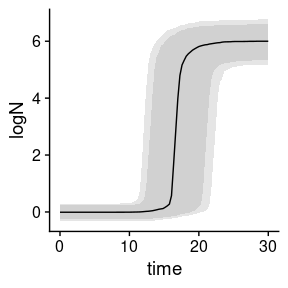In this plot, the solid line represents the mean of the simulations. Then, the two shaded areas represent, respectively, the space between the 10th and 90th, and the 5th and 95th quantiles.

By default, the function considers that there is no correlation between the model parameters. This can be varied by defining a correlation matrix. Note that the rows and columns of this matrix are defined in the order [logN0, sqrt(mu), sqrt(lambda), logNmax]. For instance, we can define a correlation of 0.7 between the square root of $$\mu$$ and the square root of $$\lambda$$:

my_cor <- matrix(c(1,   0,   0, 0,
0,   1, 0.7, 0,
0, 0.7,   1, 0,
0,   0,   0, 1),
nrow = 4)

Then, we can include it in the call to the function

stoc_growth2 <- predict_stochastic_growth(my_model, my_times, n_sims,
mean_logN0 = 0, sd_logN0 = .2,
mean_sqmu = 2,sd_sqmu = .3,
mean_sqlambda = 4, sd_sqlambda = .4,
mean_logNmax = 6, sd_logNmax = .5,
my_cor)

plot(stoc_growth2)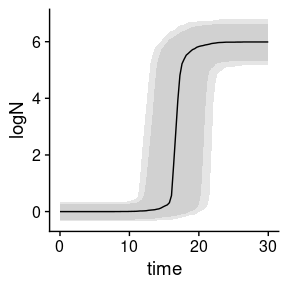Note that, in order to omit the variability/uncertainty of any model parameter, one just has to set its corresponding standard error to zero.

## One-step fitting using MCMC algorithms

Numerical algorithms based on Markov Chains have been suggested as an alternative to non-linear regression for dynamic models. For that reason, biogrowth includes the function fit_MCMC_growth() that uses the interface included in the FME package to the Adaptive Monte Carlo algorithm by Haario et al. (2006). The arguments and the requirements of this function are identical to those of fit_dynamic_growth(). The only difference is that this function has the additional argument niter, which defines the number of samples from the Markov Chain. Hence, we will repeat the previous code to define the model parameters and the data.


data("example_dynamic_growth")
data("example_env_conditions")

sec_model_names <- c(temperature = "CPM",
aw= "CPM")

known_pars <- list(Nmax = 1e4,  # Primary model
N0 = 1e0, Q0 = 1e-3,  # Initial values of the primary model
mu_opt = 4, # mu_opt of the gamma model
temperature_n = 1, temperature_xmax = 40, # Secondary model for temperature
aw_xmax = 1, aw_xmin = .9, aw_n = 1  # Secondary model for water activity
)

my_start <- list(temperature_xmin = 25,
temperature_xopt = 35,
aw_xopt = .95)

Then, we can call the fit_MCMC_growth() using these arguments plus the argument niter that we will set to 100.

my_MCMC_fit <- fit_MCMC_growth(example_dynamic_growth, example_env_conditions,
my_start,
known_pars,
sec_model_names,
niter = 100)
#> number of accepted runs: 11 out of 100 (11%)

This function returns an instance of FitDynamicGrowthMCMC with 6 entries:

• fit_results: object returned by modMCMC().
• best_prediction: an instance of DynamicGrowth with the prediction corresponding to the fitted model.
• data: data used to fit the model.
• starting: starting values used for parameter estimation.
• known: model parameters considered known.
• sec_models: type of secondary model for each environmental factor.

This class implements several S3 methods to aid in the the visualization of the results. A call to summary() returns the statistics of the Markov Chain.

summary(my_MCMC_fit)
#>      temperature_xmin temperature_xopt    aw_xopt
#> mean        27.579847        27.818451 0.95876831
#> sd           1.123397         2.623738 0.03852854
#> min         24.705271        24.042277 0.88510148
#> max         29.613602        35.275504 1.01202920
#> q025        27.752607        25.754117 0.90931392
#> q050        27.792468        25.754117 0.97325894
#> q075        27.792468        30.130946 0.97325894

And a call to plot() compares the data against the model fitted.

plot(my_MCMC_fit)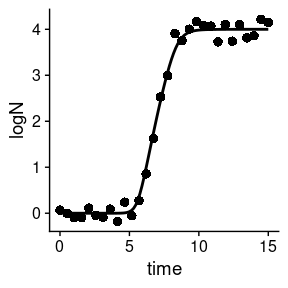As well as for fit_dynamic_growth(), the environmental conditions can be added to the plot using the add_factor argument.

plot(my_MCMC_fit, add_factor = "temperature")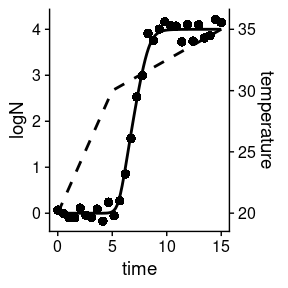## Global fitting using MCMC algorithms

Following the same logic as with the fit_MCMC_growth() function, fit_multiple_growth_MCMC() serves as an alternative to fit_multiple_growth() that uses an MCMC fitting algorithm instead of non-linear regression. The arguments of this function are identical to those of fit_multiple_growth() with the addition of niter, which defines the number of iterations from the MCMC sample.

Therefore, the definition of the data to use for the fit is identical.

data("multiple_experiments")

As well as the model definition.

## For each environmental factor, we need to defined a model

sec_names <- c(temperature = "CPM", pH = "CPM")

## Any model parameter can be fixed

known <- list(Nmax = 1e8, N0 = 1e0, Q0 = 1e-3,
temperature_n = 2, temperature_xmin = 20, temperature_xmax = 35,
pH_n = 2, pH_xmin = 5.5, pH_xmax = 7.5, pH_xopt = 6.5)

## The rest require starting values for model fitting

start <- list(mu_opt = .8, temperature_xopt = 30)

Then, the function can be called. Note that the MCMC algorithm is stochastic, so we will set the seed before fitting to grant reproducibility. Additionally, we will define upper and lower bounds for this function by passing the arguments lower and upper to modMCMC. For further ways to edit the fitting, please check the help page of modMCMC().

set.seed(12412)
global_MCMC <- fit_multiple_growth_MCMC(start, multiple_experiments, known, sec_names,
niter = 100,
lower = c(.2, 29),  # lower limits of the model parameters
upper = c(.8, 34))  # upper limits of the model parameters
#> number of accepted runs: 7 out of 100 (7%)

This function returns a list of class FitMultipleDynamicGrowthMCMC with the same entries as FitMultipleDynamicGrowth. It also implements S3 methods to inspect the parameter estimates

summary(global_MCMC)
#>          mu_opt temperature_xopt
#> mean 0.54017625       30.2407949
#> sd   0.07716531        0.9130998
#> min  0.48363806       29.2708514
#> max  0.80000000       33.4447884
#> q025 0.50000710       29.7242034
#> q050 0.52441200       30.2446953
#> q075 0.52561071       30.2446953

Or to plot the predictions of the fitted model against the data.

plot(global_MCMC)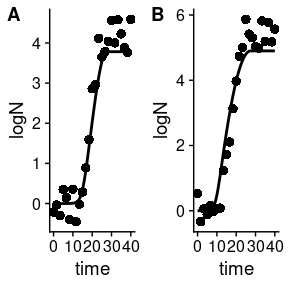Any environmental factor can be included in the plot using the add_factor argument.

plot(global_MCMC, add_factor = "temperature")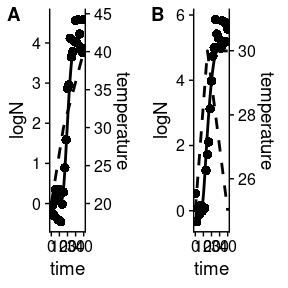## Stochastic prediction based on an MCMC fit

The function predict_MCMC_growth() provides an interface to make stochastic predictions based on parameter distributions estimated using fit_MCMC_growth() or fit_multiple_growth_MCMC(). This function has 4 arguments:

• MCMCfit an instance of FitDynamicGrowthMCMC returned by fit_MCMC_growth(), or an instance of FitMultipleGrowthMCMC returned by fit_multiple_growth_MCMC().
• times vector of time points for the calculations.
• env_conditions tibble describing the (dynamic) environmental conditions.
• niter number of samples for the Monte Carlo calculations.

For this first example, we will use the same data we used previously to illustrate the use of the fit_MCMC_growth() function. The environmental conditions were defined by example_env_conditions

example_env_conditions
#> # A tibble: 3 x 3
#>    time temperature    aw
#>   <dbl>       <dbl> <dbl>
#> 1     0          20  0.99
#> 2     5          30  0.95
#> 3    15          35  0.9

This function estimates the credible intervals based on the quantiles of the predicted microbial count at each time point. Hence, their precision depends on the number of time points and the number of simulations. If the number of time points is too low, the prediction interval would not be “smooth”. On the other hand, if the number of simulations is too low, the crecible interval would vary between repetitions of the same calculation.

As an example, we wil use 5 time points uniformly distributed between 0 and 15

my_times <- seq(0, 15, length = 5)

and 100 iterations.

niter <- 100

Once we have defined every argument, we can call the predict_MCMC_growth() function.


my_MCMC_prediction <- predict_MCMC_growth(my_MCMC_fit,
my_times,
example_env_conditions,
niter)

This function returns an instance of MCMCgrowth with 4 entries:

• sample a tibble with the sample of model parameters used for the simulations.
• simulations a tibble with the results of every individual simulation used.
• quantiles a tibble providing the calculated quantiles (5th, 10th, 50th, 90th, 95th) of the microbial count for each time point.
• model the instance of FitDynamicGrowthMCMC used for the predictions.

Hence, the individual quantiles can be retrieved from quantiles

my_MCMC_prediction$quantiles #> # A tibble: 5 x 7 #> time q50 q10 q90 q05 q95 m_logN #> <dbl> <dbl> <dbl> <dbl> <dbl> <dbl> <dbl> #> 1 0 0 0 0 0 0 0 #> 2 3.75 0 0 0.00187 0 0.00187 0.000396 #> 3 7.5 2.78 2.54 3.60 2.54 3.99 2.85 #> 4 11.2 4.00 3.99 4.00 3.99 4.00 4.00 #> 5 15 4.00 4.00 4 4.00 4 4.00 This class implements an S3 method for plot to visualize the prediction interval. plot(my_MCMC_prediction)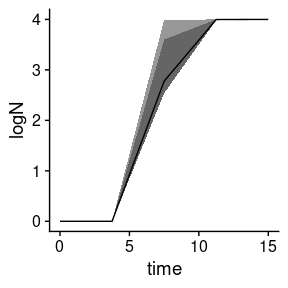In this plot, the solid line represents the median of the simulations, whereas the two shaded areas represent the space between the 10th and 90th, and 5th and 95th quantiles. As shown in this plot, the prediction interval is far from smooth. The reason for that is the low number of time points used for the calculations. Consequently, we will repeat them using 100 time points instead of 5: better_prediction <- predict_MCMC_growth(my_MCMC_fit, seq(0, 15, length = 100), example_env_conditions, niter) If we visualize the new prediction interval plot(better_prediction)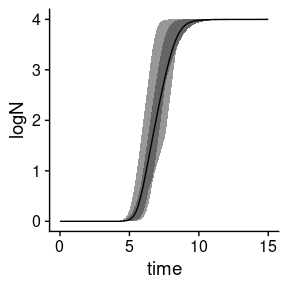it is now smoother. However, the prediction interval is still odd. Even if it is smooth, there are several inflection points that are hard to justify based on the model equations. They are the result of the low number of Monte Carlo samples used for the simulations. Hence, this number should be increased to obtain reliable intervals (not done in this vignette due to excessive compilation time). ## Distributions of times to reach a certain count For shelf-life estimation and risk assessment, the storage time required to reach a critical microbial count is usually of interest. For stochastic simulations, this time is not defined by a single value but by a probability distribution that can be estimated using the distribution_to_logcount() function. This function has two arguments: • model: an object returned by predict_stochastic_growth() or fit_MCMC_growth(). • log_count: target microbial count. For instance, we can use this function to get the distribution of times required to reach a microbial count of 4 log cFU/g based on the simulations we did before and saved in stoc_growth time_distrib <- distribution_to_logcount(stoc_growth, 4) This function returns an instance of TimeDistribution with 2 items: distribution includes a numeric vector with the distribution of times to reach log_count. summary includes summary statistics of distribution. Hence, we can observe the summary time_distrib$summary
#> # A tibble: 1 x 5
#>   m_time sd_time med_time   q10   q90
#>    <dbl>   <dbl>    <dbl> <dbl> <dbl>
#> 1   17.2    3.23     17.0  13.3  21.5

Alternatively, this class implements an S3 method to visualize the distribution of times:

plot(time_distrib)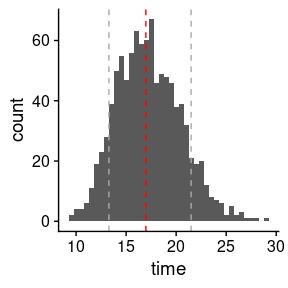In this plot, the vertical red line illustrates the median of the distribution and the grey lines the 10th and 90th quantile.